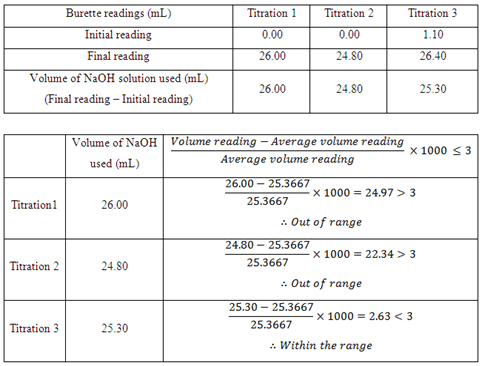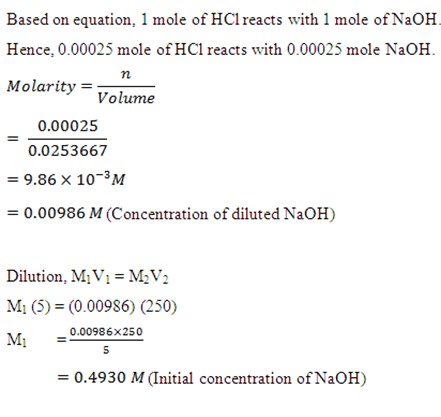## Thursday, December 15, 2011

### The Use of Volumetric Flask, Burette and Pipette in Determining the Concentration of NaOH Solution

Objectives:

1. To carry out titration of a strong acid (HCl) with a strong base (NaOH).

2. To determine the end point of titration with the use of phenolphthalein as indicator.

3. To determine the concentration of base when the concentration of acid is known by doing calculations related to titration.

Introduction:

The main purpose of this experiment is to determine the unknown concentration of a known reactant. Volumetric analysis is a method of quantitative chemical analysis that always used in titration. The technique is carried out by using a reagent of a known concentration (standard solution) and volume to react with a solution where the concentration is unknown. There are various types of titration carried out for different purposes such as acid-base titration and redox titration. Within the acid-base titration, there are four types of reactions:

(1) titration involving a strong acid and a strong base

(2) titration involving a weak acid and a strong base

(3) titration involving a strong acid and a weak base

(4) titration involving a weak acid and a weak base

The titration carried out is the acid-base titration which is based on the neutralization reaction that occurs between an acid and a base to produce salt and water. The base is added slowly into the conical flask with acid until there are all exactly reacted. This is called the end point or also known as the equivalence point of the reaction.

The equivalence point of the neutralization reaction is the point at which both acid and base have been consumed and neither is in excess. In other words, the hydrogen ion and hydroxide ion concentrations are equal in the solution. In order to determine the equivalence point in a titration, acid-base indicators need to be added to the acid solution before the titration start. The end point of a titration is indicated when the indicator changes color. An indicator is a weak organic acid or base that has distinctly different colors in its non-ionized and ionized forms. This will be discussed further in discussion section. Different indicators show different colour changes at the same pH, therefore choosing of indicator for a particular titration depends on the acid and base used.

Hydrochloric acid (HCl) and sodium hydroxide (NaOH) is used as the reactants in this experiment. HCl is a monoprotic acid which dissociate to give out one H+ ion. Monoprotic acids have acid dissociation constant, Ka, which indicates the level of dissociation in water. Hydrochloric acid has large Ka value as it is a strong acid and dissociate completely in water. NaOH is a metallic base and ionic which composed of sodium cation and hydroxide anion. The hydroxide ion makes sodium hydroxide a strong base which reacts with acids to form water and corresponding salts.

Procedure:

1. Volumetric flask was cleaned and rinsed with distilled water.
2. 25ml of NaOH solution was transferred into the volumetric flask.
3. The volumetric flask was topped up to 250ml with distilled water and rotated several times.
4. Burette was cleaned with distilled water and rinsed with 5ml NaOH solution few times and was filled.
5. Pipette was cleaned and rinsed a few times with HCl.
6. 25ml of HCl solution was pipetted into 3 conical flasks.
7. 3 drops of phenolphthalein was added into HCl solution.
8. The initial and final readings of burette were recorded.

Result and calculations:Discussion:

The titration of a strong acid and strong base in this experiment can be illustrated with a graph called a titration curve. It is a graph of pH versus volume of the solution titrated. The figure below represents the pH versus volume data of the titration curve for the HCl-NaOH titration. From the graph we may explain the chemical changes happen during titration and decide which indicators best to be used to determine the endpoint which matches the equivalence point of the neutralization.

Based on the graph, the pH has a low value at the beginning of the titration which shows the concentration of the HCl in conical flask. As the titration proceeds, the pH changes slowly until it reaches just before the equivalence point. At the equivalence point, the pH rises sharply by just adding only two drops of base. This is because when the equivalence point is reached, the number of moles of hydrogen ions and hydroxide ions is equal to each other; therefore a slight addition of base will result in a steep increase of pH. Beyond the equivalence point, the pH again rises only slowly. According to the graph, any acid-base indicator whose color changes in the pH range from about 4.0 to 10.0 is suitable for HCl-NaOH titration.

The acid-base indicator that is used for the titration of HCl-NaOH is phenolphthalein which is situated within the pH range of 8.3 to 10.0. Other indicators only have pH range within 1.0 to 8.8 which does not include the pH range beyond 9.0 as phenolphthalein where the pH range is until 10.0. Based on the interpretation of the graph, indicator whose color changes in the pH range from 4.0 to 10.0 is only suitable for the HCl-NaOH titration. Therefore phenolphthalein is chosen rather than the other indicators.

As we known an indicator is usually a weak organic acid or base that has distinctly different colour in its non-ionized and ionized forms, but what are the characteristics of an indicator that make let us determine the endpoint of a titration by showing different colours. Acid-base indicators exist in two forms, a weak acid represented as HIn and having one colour and its conjugate base represented as In- and having a different colour. The indicator does not affect the pH of the solution if only just a small amount of indicator is added to a solution. However, the ionization equilibrium of the indicator is affected by the concentration of H3O+ in the solution. When in a solution, the acid ionizes to the following ions:

Based on the Le Châtelier’s principle, increasing [H3O+] in the solution shifts the equilibrium to the left, increasing the amount of HIn and thus showing the acid colour. On the other hand, decrease in [H3O+] in a solution shifts the equilibrium to the right, increasing the amount of In- and hence displaying the base colour. In general, if 90% or more of an indicator is in the form HIn, the solution will show the acid colour. If 90% or more is in the form In-, the solution takes on the base colour. If the concentrations of HIn and In- are about equal, the indicator is in the process of changing from one form to the other and has an intermediate colour which is the mixture of acid and base colour.

Based on the results obtained, within the three titration carried out only Titration 3 is less than 3 and within the range. However, Titration 1 and 2 is more than 3 and is out of the range. The causes of the results to be out of range can be due to human errors. First of all, the NaOH solution could have been diluted as the burette used to fill the NaOH solution is rinse with distilled water and not with NaOH before use. This causes the concentration of NaOH to be slightly different from the standard solution that has been prepared. The same possibility does happen to the conical flask which is used to fill the HCl which will be titrated against NaOH where the flask only rinse with distilled water not with HCl. Thus, the concentration of HCl may be less than 0.01M. Besides that, the reading on the burette could have some minor error because during the recording of readings the meniscus shown on the burette is not clearly view. In order to correct the error, a white paper should be situated behind the burette in order to have a clearly view on the position of the meniscus so that a more accurate readings can be obtained.

Precaution steps: